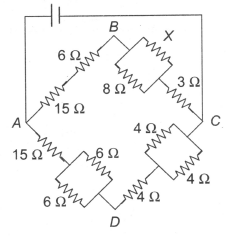In the figure given, the value of X resistance when the potential differences between B and D is zero, is

# In the figure given, the value of X resistance when the potential differences between B and D is zero, is1. A

4 ohm

2. B

6 ohm

3. C

8 ohm

4. D

9 ohm

Register to Get Free Mock Test and Study Material

+91

Verify OTP Code (required)

### Solution:

Potential difference between B and D is zero, it means Wheatstone bridge is in balanced condition.

$\mathrm{So}\text{\hspace{0.17em}}\frac{\mathrm{P}}{\mathrm{Q}}=\frac{\mathrm{R}}{\mathrm{S}}\text{\hspace{0.17em}}⇒\text{\hspace{0.17em}\hspace{0.17em}}\frac{21}{3+\text{\hspace{0.17em}}\frac{8\mathrm{X}}{\left(8+\mathrm{X}\right)}}=\frac{18}{6}\text{\hspace{0.17em}}⇒\text{\hspace{0.17em}}\mathrm{X}=8\text{\hspace{0.17em}}\mathrm{\Omega }$Register to Get Free Mock Test and Study Material

+91

Verify OTP Code (required)